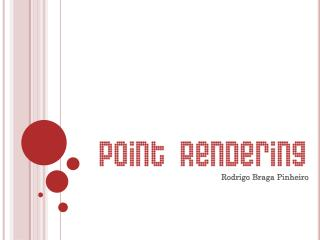DownloadDownload PresentationRodrigo Braga Pinheiro

# Rodrigo Braga Pinheiro

Télécharger la présentation## Rodrigo Braga Pinheiro

- - - - - - - - - - - - - - - - - - - - - - - - - - - E N D - - - - - - - - - - - - - - - - - - - - - - - - - - -
##### Presentation Transcript

1. Rodrigo Braga Pinheiro

2. What is it? It is a point-based rendering, i.e., the 3D models are represented by points (point cloud) and not by triangles, as they are commonly represented. Point cloud - Set of vertices in a 3D coordinate system. These vertices are usually defined by X, Y, and Z coordinates, and typically are intended to be representative of the external surface of an object.

3. Motivation • Evolution of point acquisition’s devices. • Best efficiency when compared with rendering based on triangles or another primitive that needs information about connectivity of points. • High quality (with efficiency) of rendering when the point cloud is dense. • Hardware implementation is possible.

4. Pipeline

5. 3D acquisition (Scanner) • 3D Scanning - A scan is taken at some viewpoint. • View planning - A decision is made about where to position the scanner relative to the object in order to perform the next scan. • Registration - After all scans have been acquired, a global registration algorithm may be used to simultaneously minimize misalignment errors over all pairs of overlapping scans. • Merging - The aligned scans frequently contain signiﬁcant regions in which many scans overlap. Merging these logically separate scans into a single model both reduces storage and averages away some of the scanning noise. • Post-processing - Depending on the ﬁnal use for the model, the output of the merging step may undergo further processing, including noise or outlier reduction, automated ﬁlling of small remaining holes, and curvature-adaptive resampling or decimation.

6. 3D acquisition (Scanner) • Triangulation-based Triangulation-based 3D scanners ﬁnd the positions of points on a surface by computing corresponding pixels from two viewpoints. The correspondence deﬁnes a pair of rays in space, and the intersection of the rays determines a 3D position.

7. 3D acquisition (Scanner) • Passive Stereo • Active Stereo • Structured Light • Light Stripe • Multiview Triangulation and Structure from Motion • Pulsed and Modulated Time of Flight • Shape from Shading and Photometric Stereo

8. 3D acquisition (Photography)

9. Spherical Flipping Consider a D-dimensional sphere with radius R, centered at the origin (C), and constrained to include all the points in P. Spherical flipping reflects a point ϵP with respect to the sphere (spherical mirror) by applying the following equation: where: = point inside the sphere R = radius of sphere

10. Spherical Flipping Intuitively, spherical flipping reflects every point internal to the sphere along the ray from C to toits image outside the sphere. Figure 1 Figure 2 Figure 2 - Spherical flipping (in red) of a 2D curve (in blue) using a sphere (in green) centered at the view point (in magenta).

11. Convex Hull The convex hull of a set Q of points is the smallest convex polygon P for which each point Q is either on the boundary of P or in its interior.

12. Convex Hull (3D) • 3rd Party library

13. Convex Hull • Denote by the transformed point cloud of P: • Calculate the convex hull of U {C}, i.e., the set that contains the transformed point cloud AND the center of the sphere.

14. Point Selection • A point is marked visible from C if its inverted point lies on the convex hull of U {C}.

15. Before

16. After

17. References • Point-Based Graphics (The Morgan Kaufmann Series in Computer Graphics) • MarkusGross, HanspeterPfister • Direct Visibility of Point Sets • SagiKatz, Ayellet Tal, Ronen Basri • Mesh segmentation using feature point and core extraction • SagiKatz, George Leifman, Ayellet Tal • Introduction to Algorithms • Thomas H. Cormen, Charles E. Leiserson, Ronald L. Rivest, Clifford Stein

18. Point Selection (Prove) Result of spherical flipping The shape of L for different values of β(in degrees), where = (10;0), R = 30.

19. Point Selection (Prove) • L and the X-axis define the empty region associated with . ”How much” is visible, depends on the size of the region. • For every given point , there exist two special points on either side of P, and ϵP. The region bounded between the curves in the “L equation”, from through and from through , is the largest possible empty region.

20. Point Selection (Prove) From “L Equation”, it can be deduced that the largest region corresponds to the smallest β. This means that βj and βk that correspond to the largest possible empty region, are the smallest possible for . Note that βjand βk can be extracted from “L Equation”. For to be visible, the sum of βjand βk should satisfy βj + βk = const (i.e., a large empty region is associated with ) The empty gray region between Lj and Lk, as defined by the values of βj + βk.

21. Point Selection (Prove)